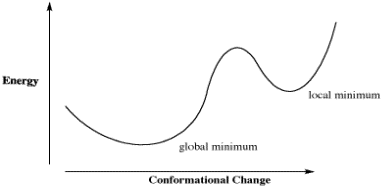# CA5. Molecular Modeling

### CA5. Molecular Modeling

The shapes of molecules can be predicted by tallying up estimates of steric and torsional interactions. This task is referred to as a molecular mechanics calculation. The basis set used may vary from the one given here, but the idea is the same: a limited set of information is applied to molecules, no matter how large or complex, in order to measure the relative energy of different conformations. Typically a calculation is followed by a change in a dihedral angle somewhere, the calculation is repeated and so on, so that eventually the most stable conformation is found.

A calculation like this could take a very long time. Even on a computer, there is usually a need to speed things up. A common short cut for employs the following approach. If a change in dihedral angle results in a decrease in energy, the calculation continues with a new change in that dihedral angle. Eventually, if changes lead to increased energy, the program assumes the lowest energy has already been passed and it backtracks to that point again. It may then try another dihedral using the same method. In this way the computer doesn't waste time on calculations involving high energy eclipsed conformations or ones with steric problems. As a result, a molecular mechanics calculation can usually estimate the lowest energy conformer in a few seconds.

The limitation of that approach can be seen in a generic plot of energy vs. conformational change in which there is more than one low-energy conformer. How does the calculation determine that it has found the absolute lowest energy conformer, and not just a dip in the energy surface?Figure CA5.1. Plot of energy vs. conformational change for a fictional molecule.

For example, in a plot of butane's relative energy vs its dihedral angle around C2-C3. The gauche conformation occupies a "local energy minimum", meaning that the energy is lower here than when the dihedral angle is either greater or smaller, because in either direction the molecule would arrive at an eclipsed conformation with higher energy. In the approach described above, typically the default approach in most calculations, the computer could get stuck at the gauche conformation, because a change in either direction results in an increase in energy. However, if the computer would just push past those energy maxima then it would arrive at the anti conformation, which is the "global energy minimum", with the lowest energy of all.

• Beware of local minima when looking for the most stable conformer on a computer.
• Usually, you can tell whether the computer has the right answer based on your understanding of sterics.

Complete conformational analysis sometimes requires some method of "dihedral angle driving". In this kind of approach, the software user can tell the computer to calculate energies a given number of times around a given dihedral angle; for example, maybe a calculation would be done every sixty degrees for the dihedral around the C2-C3 bond in butane, or maybe every thirty degrees. That way, the computer is sure to find the global minimum for a particular dihedral angle.

More advanced methods may be required to arrive at correct low-energy structures for more complicated molecules. For example, hydrogen bonding is clearly a major factor controlling the structure of molecules such as DNA and proteins. We have looked only at steric and torsional strains because they are ubiquitous in molecular mechanics, but different basis sets, and different types of calculations, could take into account additional interactions such as hydrogen bonding or other electronic phenomena which would help correctly establish the shapes of more complex molecules.